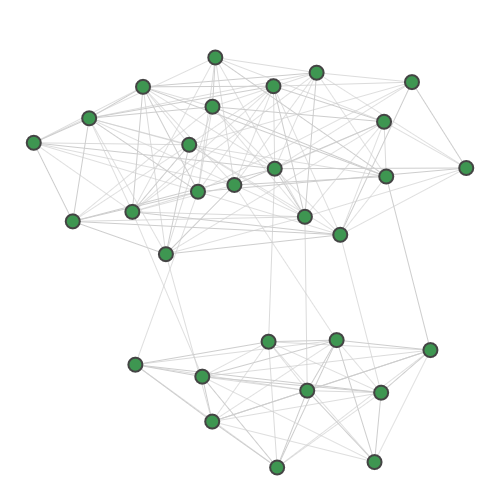# sf.apps.data.Planted¶

class Planted[source]

A random 30-node graph containing a dense 10-node subgraph planted inside .

The graph is generated by joining two Erdős–Rényi random graphs. The first 20-node graph is generated with edge probability of 0.5 and the second 10-node planted graph is generated with edge probability of 0.875. The two graphs are joined by selecting 8 vertices at random from both and adding an edge between them.

The 10-node planted clique is contained within the final 10 nodes of the graph.

Graph:n_mean = 8
threshold = True
n_samples = 50000
modes = 30
n_mean = 8
threshold = True
 counts([axis]) Count number of photons or clicks.
counts(axis=1)

Count number of photons or clicks.

Counts number of photons/clicks in each sample (axis==1) or number of photons/clicks in each mode compounded over all samples (axis==0).

Parameters

axis (int) – axis to perform count

Returns

counts from samples

Return type

list